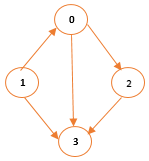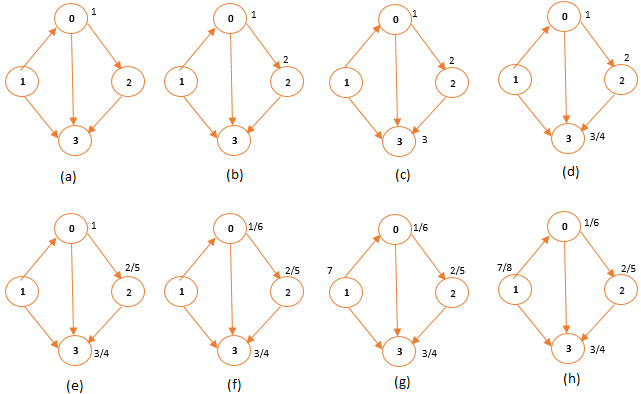# Implementation and Analysis of Depth First Search(DFS)

 Category: Data Structure And Algorithms Tags:Depth First Search Code Files

## Introduction

We have already learnt about graphs and their representation in Adjacency List and Adjacency Matrix as well we explored Breadth First Search (BFS) in our previous article. In this article we are going to explore Depth First Search (DFS) which also is used as graph search algorithm. Unlike BFS, DFS goes in depth and from there it backtracks the graph. DFS keeps two timestamp properties discovered time and finish time. When a vertex is discovered it assigns discovered time and assigns finish time once vertex is processed. We will use directed graph this time which is shown below:Fig 1: Directed Graph

Above we can see a directed graph which keeps directions from one vertex to other, we can make adjacency list of above graph:
0 -> 2 -> 3
1 -> 3 -> 0
2 -> 3

## Implementation

First we will create vertex structure given below:

```/// <summary>
/// Vertex class
/// </summary>
public class Vert
{
public int Vertex { get; set; }
public Vert Next { get; set; }
public Vert(int val)
{
Vertex = val;
}

public Vert Parent { get; set; }
public int D { get; set; }  //discovered time
public int F { get; set; }  //finished time
public char Color { get; set; } = 'W';
}
```

Above we created vertex which has all required properties unlike BFS it doesn’t have distance instead it has discover time(D) and finish time(F). Now we will create class DepthFirstSearch:

```public class DepthFirstSearch
{
int time = 0;
public DepthFirstSearch(int totalVertix)
{
for (int i = 0; i < adjList.Length; i++)
}
/// <summary>
/// </summary>
/// <param name="u">vertex where v has to be linked</param>
/// <param name="v">vertex which has to be linked</param>
public void AddV(int u, int v)
{
while (tempU.Next != null)
{
if (tempU.Vertex != v)
tempU = tempU.Next;
else
return;
}
tempU.Next = new Vert(v);
}
public void DFS() {...}
void DFS_Visit(Vert u) {...}
public void PrintPropertiesOfAllVertex() {...}
}
```

AddV method adds vertex in adjacency list you will find it a bit different than we had in BFS because we have to create directed graph here but in BFS we created undirected graph. Methods DFS, DFS_Visit and PrintPropertiesOfAllVertex are used to search and print, now we will see implementation of DFS:

```/// <summary>
/// Discovers vertex using DFS
/// </summary>
public void DFS()
{
for (int i = 0; i < adjList.Length; i++)
{
u.Color = 'W';  //coloring all white
u.Parent = null;
}

for (int i = 0; i < adjList.Length; i++)  //routing all vertex in graph
{
if (u.Color == 'W')
{
DFS_Visit(u);
}
}
}
```

Above we setting color white to all the vertices in adjacency list and sending these to get processed one by one if color is white, main work is done by DFS_Visit method:

```/// <summary>
/// Processing a discovered vertex
/// </summary>
/// <param name="u">discovered vertex</param>
void DFS_Visit(Vert u)
{
time++;
u.Color = 'G';  //graying
u.D = time;     //setting discovered time
Vert v = u.Next;

while (v != null)
{
if (mainV.Color == 'W')
{
mainV.Parent = u;  //setting parent
DFS_Visit(mainV);  //resursive
}
v = v.Next;
}

u.Color = 'B';  //blacking
time++;
u.F = time;    //setting finish time
}
```

We traverse through adjacency list in order to find all vertices connected to a vertex. We gray vertex u and recursively process all connected vertices, we also assign discovered time and finish time. Now let’s see method PrintPropertiesOfAllVertex which actually prints vertex discovered time and finish time:

```/// <summary>
/// Prints vertices after processing
/// </summary>
public void PrintPropertiesOfAllVertex()
{
{
Console.WriteLine("Vertex {0} discover time: {1} and finish time {2}", v.Vertex, v.D, v.F);
}
}
```

We have already seen PrintPath method in BFS, same we can implement here to find path between source and destination. Now let’s execute above code from Main method:

```static void Main(string[] args){    DepthFirstSearch dfs = new DepthFirstSearch(4);    dfs.AddV(0, 2);    dfs.AddV(1, 3);    dfs.AddV(1, 0);    dfs.AddV(0, 3);    dfs.AddV(2, 3);    dfs.DFS();    dfs.PrintPropertiesOfAllVertex();    Console.ReadLine();}
```

Above we added vertex in adjacency list and called method DFS which will discover vertex and PrintPropertiesOfAllVertex will print all vertices properties after DFS call. Output will be:

Vertex 0 discover time: 1 and finish time 6
Vertex 1 discover time: 7 and finish time 8
Vertex 2 discover time: 2 and finish time 5
Vertex 3 discover time: 3 and finish time 4

You can see in below image how DFS traverse through a graph and assigns discover time and finish time which are important properties to analyze a graph and can be used in parenthesis theorem (parenthesis theorem states if you replace discovered time of a vertex with left parenthesis "(" and finish time with right parenthesis ")" then it will be well formed expression).Fig 2: Depth First Search Traversing and calculation of discovered and finish time

 Like0 PeopleNikhil Joshi Ceo & Founder at Dotnetlovers Atricles: 158 Questions: 16 Given Best Solutions: 16 *

Login via:x